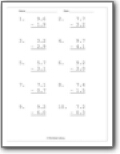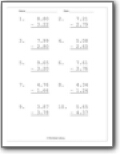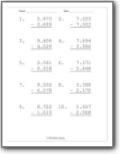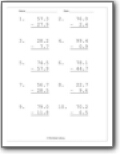# Decimal Subtraction Worksheets

#### How to Subtract Decimals

Now that you've learned how to add decimals, the next step is to learn how to subtract. Also, it would help if you were extra careful in solving these questions. Let's take an example of 45.3 - 2.7.
45.3
-   2.7

42.6

As you notice, three are less than 7; so we need to borrow one from the adjacent number to make it greater by rules. And, then we start to perform operations. Keep in mind that we have to repeat the same steps until all numbers are resolved.###### Tenths

Students will subtract two values that together that have a ones and tenths value.###### Hundredths Place

You will subtract and find the product of two values that have a ones, tenths, and hundredths value.###### Thousandths

Following this pattern, we looks determine the final difference of values that include a thousandths place.###### Subtract Tens, Ones, and Tenths

You will subtract two values that have all these place values.